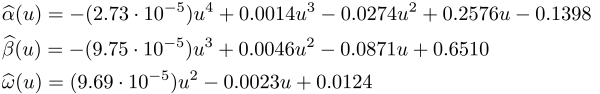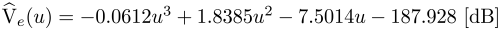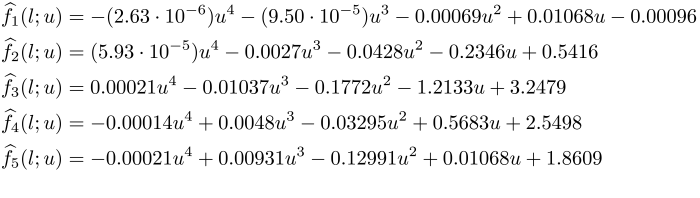# International Audio Laboratories Erlangen

## Simulating wind noise with airflow speed-dependent characteristics

D. Mirabilii1, A. Lodermeyer1, F. Czwielong2, S. Becker2 and E. A. P. Habets1

1 International Audio Laboratories Erlangen
2 Aerodynamics and Acoustics, Institute of Fluid Mechanics, FAU Erlangen-Nürnberg

Accepted in the Proc. Intl. Workshop Acoust. Signal Enhancement (IWAENC), Bamberg 2022

### Abstract

Generating noise samples is crucial in developing and testing noise reduction algorithms or training deep learning models. This work proposes a wind noise generation model with airflow speed-dependent features. A linear predictive analysis of wind noise measured in a wind tunnel at different flow velocities was carried out. This analysis showed that temporal and spectral features depend on the flow speed. The prediction residual's statistics and the filter coefficients are first extracted and then modeled based on the flow speed. The obtained models are then combined to synthetically generate wind noise given a time-varying flow speed profile as input, in contrast to an existing framework where temporal and spectral features were assumed speed-invariant. A subjective evaluation is carried out to assess the perceptual authenticity of the generated noise compared to the existing method.

### Experimental setupFigure 1: Experimental setup in the aeroacoustic wind tunnel. The microphone array was connected to a turntable through a shaft. The rotation is shown in the video below.

Video 1: Microphone array rotation and audio recording. This operation was repeated for each flow speed (from 2 to 18 m/s).

### Block scheme of the methodFigure 2: Block scheme of the proposed wind noise generation model.

### Model parameters

Short-term variance model GARCH(1,1)Long-term variance modelFilter model (LSF)To assess the adequacy of the models, we computed the Akaike Information Criterion (AIC) and the Bayesian Information Criterion (BIC) from a collection of models obtained via polynomial regression with an increasing order. The independent variable was the flow speed. The dataset was split 50% between estimation and validation sets, i.e., each audio sample was divided in half. The model selection was then performed individually for each quantity (short-term variance, long-term variance, and individual LSF coefficients). For each model, the regression order was selected based on the one yielding the minimum AIC and BIC scores computed on the validation set.

### Audio Examples

The following audio examples are the outcome of different wind noise generation models.

• Baseline: flow speed-invariant LPC coefficients, three-state Markov model as long-term gain, and white noise as short-term gain [1-2].
• WHITE-variable lpc: flow speed-dependent LPC coefficients and long-term gain, and white noise as short-term gain
• GARCH-fixed lpc: flow speed-invariant LPC coefficients, flow speed-dependent long-term gain, and GARCH model as short-term gain
• GARCH-variable lpc: flow speed-dependent LPC coefficients and long-term gain, and GARCH model as short-term gain

The top graphs show the generated wind noise waveform. The middle graphs show the associated spectrogram (FFT length of 512 with 50% overlap). The bottom graphs show the wind activity for the Baseline (n = no wind, l = low wind, h = high wind) or the wind speed for the remaining models. The proposed models share the same wind speed profile generated by sampling from a two-parameter Weibull distribution.

The samples generated with the proposed models were downsampled from 48 kHz to 16 kHz for a fair comparison to the baseline model. The original MATLAB implementation of the Baseline can be found here.Note: Please use Chrome or Safari for optimal audio/video synchronization. If you encounter playback problems, please reload the page.

### References

 C. M. Nelke and P. Vary, “Measurement, analysis and simulation of wind noise signals for mobile communication devices,” in the Proc. pf the International Workshop on Acoustic Signal Enhancement (IWAENC), pp. 327–331, 2014.

 C. M. Nelke, "Wind Noise Reduction – Signal Processing Concepts", Ph.D. thesis, RWTH Aachen University, 2016.